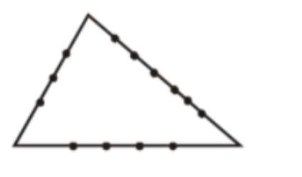# If the sides AB, BC andQuestion:

If the sides $\mathrm{AB}, \mathrm{BC}$ and $\mathrm{CA}$ of a triangle $\mathrm{ABC}$ have 3,5 and 6 interior points respectively, then the total number of triangles that can be constructed using these points as vertices, is equal to :

1. (1) 364

2. (2) 240

3. (3) 333

4. (4) 360

Correct Option: , 3

Solution:Total Number of triangles formed $={ }^{14} \mathrm{C}_{3}-{ }^{3} \mathrm{C}_{3}-{ }^{5} \mathrm{C}_{3}-{ }^{6} \mathrm{C}_{3}$

$=333$